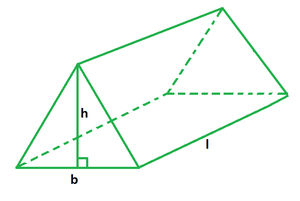Open in App
Not now

# Volume of a Triangular Prism Formula

• Last Updated : 29 Sep, 2022

A prism is a three-dimensional solid figure with two identical ends. It is made up of flat sides, similar bases, and equal cross-sections. Its faces are parallelograms or rectangles without bases. Such a prism that has three rectangular faces and two parallel triangle bases is called a triangular prism. The triangular bases are connected by lateral faces that run parallel to one another.### Volume of a Triangular Prism Formula

A triangular prism’s volume is defined as the space inside it or the space filled by it. Knowing the base area and height of a triangular prism is all that is required to calculate its volume. The volume of a triangular prism is equal to the product of the base’s area and the prism’s height, also known as the length of the prism. The base area of a triangular prism is equal to half of the product of the triangular base and its altitude.

Formula

V = (1/2) × b × h × l

where,

b is the triangular base,

h is the altitude of the prism,

l is the length of prism.

### Sample Problems

Problem 1. Find the volume of a triangular prism if its base is 6 cm, altitude is 8 cm and length is 12 cm.

Solution:

We have, b = 6, h = 8 and l = 12.

Using the formula we have,

V = (1/2) × b × h × l

= (1/2) × 6 × 8 × 12

= 3 × 8 × 12

= 288 cu. cm

Problem 2. Find the volume of a triangular prism if its base is 5 cm, altitude is 7 cm and length is 8 cm.

Solution:

We have, b = 5, h = 7 and l = 8.

Using the formula we have,

V = (1/2) × b × h × l

= (1/2) × 5 × 7 × 8

= 5 × 7 × 4

= 140 cu. cm

Problem 3. Find the length of the triangular prism if its base is 6 cm, altitude is 9 cm and volume is 98 cu. cm.

Solution:

We have, b = 6, h = 9 and V = 98.

Using the formula we have,

V = (1/2) × b × h × l

=> 98 = (1/2) × 6 × 9 × l

=> 196 = 27l

=> l = 196/27

=> l = 7.25 cm

Problem 4. Find the altitude of the triangular prism if its base is 8 cm, length is 14 cm and volume is 504 cu. cm.

Solution:

We have, b = 8, l = 14 and V = 504.

Using the formula we have,

V = (1/2) × b × h × l

=> 504 = (1/2) × 8 × h × 14

=> 504 = 56h

=> h = 504/56

=> h = 9 cm

Problem 5. Find the area of the base of the triangular prism if its length is 18 cm, height is 10 cm and volume is 450 cu. cm.

Solution:

We have, l = 18, h = 10 and V = 450.

Using the formula for volume we have,

V = (1/2) × b × h × l

=> 450 = (1/2) × b × 10 × 18

=> 450 = 90b

=> b = 450/90

=> b = 5 cm

Hence, the area of triangular base is,

A = (1/2) × b × h

= (1/2) × 5 × 10

= 25 sq. cm

My Personal Notes arrow_drop_up